Test: Properties of Soil - 1

# Test: Properties of Soil - 1

Test Description

## 20 Questions MCQ Test Soil Mechanics | Test: Properties of Soil - 1

Test: Properties of Soil - 1 for Civil Engineering (CE) 2023 is part of Soil Mechanics preparation. The Test: Properties of Soil - 1 questions and answers have been prepared according to the Civil Engineering (CE) exam syllabus.The Test: Properties of Soil - 1 MCQs are made for Civil Engineering (CE) 2023 Exam. Find important definitions, questions, notes, meanings, examples, exercises, MCQs and online tests for Test: Properties of Soil - 1 below.
Solutions of Test: Properties of Soil - 1 questions in English are available as part of our Soil Mechanics for Civil Engineering (CE) & Test: Properties of Soil - 1 solutions in Hindi for Soil Mechanics course. Download more important topics, notes, lectures and mock test series for Civil Engineering (CE) Exam by signing up for free. Attempt Test: Properties of Soil - 1 | 20 questions in 60 minutes | Mock test for Civil Engineering (CE) preparation | Free important questions MCQ to study Soil Mechanics for Civil Engineering (CE) Exam | Download free PDF with solutions
 1 Crore+ students have signed up on EduRev. Have you?
Test: Properties of Soil - 1 - Question 1

### Liquid limit of a soil indicates its

Detailed Solution for Test: Properties of Soil - 1 - Question 1

The liquid limit of soil is the percentage of water content at which the soil passes from the plastic state to the liquid state or the Liquid limit indicates how much water the soil can hold without getting into the “liquid” state. The compressibility of soil is indicated by liquid limit, i.e. the compressibility of the soil generally increases with an increase in the liquid limit.

Test: Properties of Soil - 1 - Question 2

### An undisturbed soil sample has a plastic limit of 25%, a natural moisture content of 40% and liquidity index of 50%, Its liquid limit in % will be

Detailed Solution for Test: Properties of Soil - 1 - Question 2

The formula for liquidity index is given as follows where w is the natural moisture content, wp is the plastic limit, Ip is the liquid limit. So we can calculate the liquid limit by substituting the values.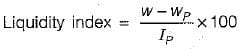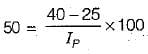⇒ Ip = 30%
Also Ip = wl - wp
⇒ Wl = 30 + 25 = 55%

Test: Properties of Soil - 1 - Question 3

### A well graded soil has a coefficient of curvature between

Detailed Solution for Test: Properties of Soil - 1 - Question 3
• The coefficient of curvature should lie between 1 and 3 for well-graded soil.
• The coefficient of gradation is also known as the coefficient of curvature and it is given by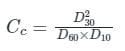• The slope of the gradation curve of the soil gives the coefficient of curvature which represents the shape of the particle size distribution curve.
Test: Properties of Soil - 1 - Question 4

The ratio of the undisturbed shear strength to the remoulded shear strength in cohesive soil under undrained conditions is

Detailed Solution for Test: Properties of Soil - 1 - Question 4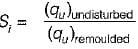at unaltered water condition i.e. at undrained condition. Remoulded shear strength is less than undisturbed shear strength.

Test: Properties of Soil - 1 - Question 5

Number of phases in soil mass is:

Detailed Solution for Test: Properties of Soil - 1 - Question 5

Soil has three phase representation as follows,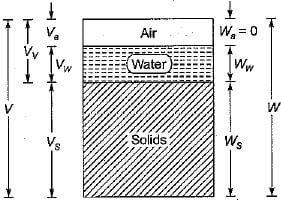Test: Properties of Soil - 1 - Question 6

Which of the following is a measure of particle size range?

Detailed Solution for Test: Properties of Soil - 1 - Question 6

The uniformity of a soil is expressed qualitatively by a term known as uniformity coefficient, Cu, given by,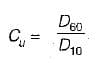The larger the numerical value of Cu, the more is the range of particles.

Test: Properties of Soil - 1 - Question 7

The ratio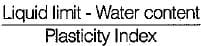for a soil mass is called:

Detailed Solution for Test: Properties of Soil - 1 - Question 7

The consistency index indicates the consistency (firmness) of a soil. It shows the nearness of the water content of the soil to its plastic limit. A soil with a consistency index of zero is at the liquid limit. It is extremely soft and has negligible shear strength. On the other hand, a soil at a water content equal to the plastic limit has a consistency index of 100%, indicating that the soil is relatively firm.

Test: Properties of Soil - 1 - Question 8

The principle involved in the relation
γsub. = γsat - γw is

Detailed Solution for Test: Properties of Soil - 1 - Question 8

When the soil exists below Water, it is in a submerged condition. When a volume  V of soil is sub merged in water, then according to Archimede’s principle it displaces an equal volume of water. Thus the net mass of soil when submerged is reduced.

Test: Properties of Soil - 1 - Question 9

The uniformity coefficient of soil is given as

Detailed Solution for Test: Properties of Soil - 1 - Question 9
• The uniformity coefficient (Cu) is defined as the ratio of D60 to D10.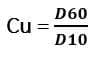• A value of Cu greater than 4 to 6 classifies the soil as well graded.
• When Cu is less than 4, it is classified as poorly graded or uniformly graded soil
• Uniformly graded soil has identical particles with Cu value approximately equal to 1.
• A uniformity coefficient value of 2 or 3 classifies the soil as poorly graded. Beach sand comes under this category.
• Higher value of Cu indicates that the soil mass consists of soil particles with different size ranges.
Test: Properties of Soil - 1 - Question 10

Given for a soil sample:
Degree of saturation = 90%
Specific gravity of soil grains = 2.70
Void ratio = 0.30
The water content of the sample is

Detailed Solution for Test: Properties of Soil - 1 - Question 10

The formula for water content is written as follows, where w = water content, G = Specific Gravity of soil grains, S = Degree of Saturation and e = Void ratio.
Gw = Se

We can calculate the value of 'w' by substituting the values of G, S and e.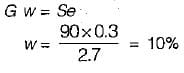Test: Properties of Soil - 1 - Question 11

For distinguishing clays from silts in the field, a moist soil is rolled into a thread of 3 mm diameter. This test will indicate the

Detailed Solution for Test: Properties of Soil - 1 - Question 11
• 1 cm2 of specimen of soil is taken and moistened if necessary and is rolled on a smooth glass plate to about 3mm diameter (plastic limit test).
• If crumbling does not occur, fold the thread, knead and roll again until the moisture of the soil reduces by drying during manipulation to plastic limit, which is indicated by crumbling that occurs during rolling.
• Characteristics of thread approaching plastic limit offers means of identification of soil.
• Plastic clays can be easily rolled and its plasticity can be easily found but if soil contains much silt, it can’t be rolled or becomes difficult to roll.
Test: Properties of Soil - 1 - Question 12

Which one of the following is the water content of the mixed soil made from 1 kg of soil (say A) with water content of 100% and 1 kg of soil (say B) with water content of 50%?

Detailed Solution for Test: Properties of Soil - 1 - Question 12

Water content,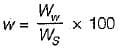Total weight,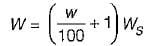Weight of solids in soil A,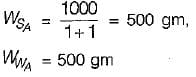Weight of solids in soil B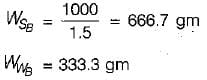In mixed soil,
Ww = 500 + 333.3 = 833.3 gm
Ws = 500 + 666.7 = 1166.7 gm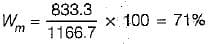Test: Properties of Soil - 1 - Question 13

Which one of the following gives the correct decreasing order of the densities of a soil sample?

Detailed Solution for Test: Properties of Soil - 1 - Question 13

We know that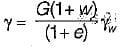For dry soil, w = 0;
For saturated soil, w = ws
and for Wet soil 0 < w < ws
Thus, dry density < wet density < saturated density.
Now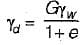and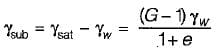Thus submerged density < dry density

Test: Properties of Soil - 1 - Question 14

Match List-I with List-ll and select the correct answer using the codes given below in the options: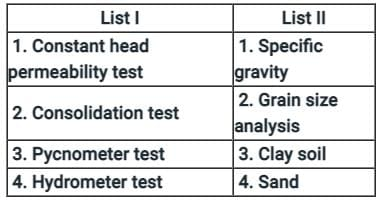Detailed Solution for Test: Properties of Soil - 1 - Question 14

The coefficient of permeability for coarse-grained soil (Gravel and sand) is determined by means of the Constant-head permeability test. The degree of saturation of soil should be 100%.
By Darcy’s Law,

Q = k i A

Q=khA/L

k=QL/Ah

where, q = Discharge collected in time ‘t’, L = Distance between manometer taping points, A = Cross-sectional area of the sample, H = Difference in manometer levels i.e the head loss.

2. Consolidation Test:

(i) This is used to determine the rate and magnitude of soil consolidation when the soil is restrained laterally and loaded axially. This test is conducted by an Oedometer. As disturbing specimens have a certain pre-consolidation pressure, so for conducting the consolidation test undisturbed soil specimen is required.

(ii) Oedometer is used to find out the void ratio at the end of various stress levels and hence curve is plotted. This curve is useful in calculating the settlement of the soil layer.

(iii) Consolidation test is generally performed on clay soil.

3. Pycnometer test is used to determine the specific gravity of cohesion-less soils and water content.

4. Hydrometer test:

• Measuring the particle size distribution(Grain Size Analysis) of fine-grained soils like clay and silt is best performed using the soil hydrometer test
• The hydrometer analysis of soil, based on Stokes’ law, calculates the size of soil particles from the speed at which they settle out of suspension from a liquid.
Test: Properties of Soil - 1 - Question 15

For a given soil sample,
If Cc = 1.0 and Cu = 4.0, then the value of D30/D10 would be

Detailed Solution for Test: Properties of Soil - 1 - Question 15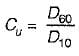and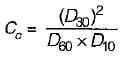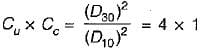⇒ D30/D10 = 2

Test: Properties of Soil - 1 - Question 16

At liquid limit, all soils possess

Detailed Solution for Test: Properties of Soil - 1 - Question 16

Liquid limit:

It is the water content corresponding to the arbitrary limit between liquid and plastic state of consistency of soil.

It is defined as the minimum water content at which the soil is still in the liquid state, but has infinitesimal resistance against flow which can be measured by any standardised procedure.

With reference to the standard liquid limit device, it is defined as the minimum water content at which a part of soil cut by a groove of standard dimensions, will flow together for a distance of 12 mm under an impact of 25 blows in the device.
Other Important points:

Plastic limit: It is defined as the minimum water content at which a soil will just begin to crumble when rolled into a thread approximately 3 mm in diameter.

Shrinkage limit: It is defined as the maximum water content at which a reduction in water content will not cause a decrease in the volume of a soil mass. It is the lowest water content at which a soil can still be completely saturated.

Test: Properties of Soil - 1 - Question 17

Which of the following methods is most accurate for the determination of the water content of soil?

Detailed Solution for Test: Properties of Soil - 1 - Question 17

Oven dry method is the most accurate and simplest method for water content determination.

In this method complete drying of soil sample occur and water content in sample is calculated accurately by a maintained temperature in the oven ( 105° C to 110° C) for 24 hours.

Note:

For highly organic soils a low temperature of about 60° C is preferable.

If Gypsum is present, the temperature should not be more than 80° C but for a long time.

Radiation method is based on energy loss during radio active isotropes material (E.g. cobalt-60) emmites on one end and received by detector on other end. This is an approximate method.

In calcium carbide method, Acetylene gas exerts pressure on the pressure gauge which indicate the water content present in soil sample. This is not an accurate method since no control over chemical reactions.

Sand bath method is field method and there is no control over heat given to soil sample. Therefore, it is not suitable for organic soil and soil having higher gypsum content.

Test: Properties of Soil - 1 - Question 18

A pycnometer is used to determine

Detailed Solution for Test: Properties of Soil - 1 - Question 18

Pycnometer test is used to determine the specific gravity of cohesion less soils and water content.

Dry density or in-situ unit weight is determined by using the following methods:

1. Sand Replacement

2. Core-Cutter

3. Water Displacement

Other methods to determine the water content are:

1. Oven Drying Method

2. Calcium Carbide/Rapid Moisture

3. Sand Bath Method

5. Torsion Balance Moisture Meter

Test: Properties of Soil - 1 - Question 19

In hydrometer analysis for a soil mass

Detailed Solution for Test: Properties of Soil - 1 - Question 19

The grain size distribution of fine soil is determined using sedimentation analysis. Sedimentation analysis is performed using two methods:

1) Pipette method

2) Hydrometer method.

A hydrometer is a device used to measure the specific gravity of liquids.

The following three corrections are necessary for :

1. Meniscus correction: Since the hydrometer readings increase downward on the stem, the meniscus correction (Cm) is always positive.

2. Temperature correction: If the temperature at the time of the test is more than that of calibration of the hydrometer, the observed reading will be less and the correction (Ct) would be positive and vice versa.

3. Deflocculating agent correction: The addition of the deflocculating agent increases the density of the suspension and thus a correction (Cd) is applied which is always negative.

R = Rh' + cm ± Ct - Cd
Cm = Meniscus correction
Ct - Temperature correction
Cd = Dispersive agent correction

Test: Properties of Soil - 1 - Question 20

Valid range for S, the degree of saturation of soil in percentage is

Detailed Solution for Test: Properties of Soil - 1 - Question 20

Degree of saturation represents the portion of volume of voids which is filled with water i.e.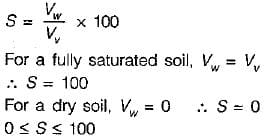## Soil Mechanics

30 videos|50 docs|66 tests
 Use Code STAYHOME200 and get INR 200 additional OFF Use Coupon Code
Information about Test: Properties of Soil - 1 Page
In this test you can find the Exam questions for Test: Properties of Soil - 1 solved & explained in the simplest way possible. Besides giving Questions and answers for Test: Properties of Soil - 1, EduRev gives you an ample number of Online tests for practice

## Soil Mechanics

30 videos|50 docs|66 tests